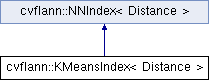OpenCV  3.4.5 Open Source Computer Vision
cvflann::KMeansIndex< Distance > Class Template Reference

#include "kmeans_index.h"

Inheritance diagram for cvflann::KMeansIndex< Distance >:## Classes

class  KMeansDistanceComputer

## Public Types

typedef void(KMeansIndex::* centersAlgFunction) (int, int *, int, int *, int &)

typedef Distance::ResultType DistanceType

typedef Distance::ElementType ElementType

## Public Member Functions

KMeansIndex (const Matrix< ElementType > &inputData, const IndexParams &params=KMeansIndexParams(), Distance d=Distance())

KMeansIndex (const KMeansIndex &)

virtual ~KMeansIndex ()

void buildIndex () CV_OVERRIDE

void chooseCentersGonzales (int k, int *indices, int indices_length, int *centers, int &centers_length)

void chooseCentersKMeanspp (int k, int *indices, int indices_length, int *centers, int &centers_length)

void chooseCentersRandom (int k, int *indices, int indices_length, int *centers, int &centers_length)

void findNeighbors (ResultSet< DistanceType > &result, const ElementType *vec, const SearchParams &searchParams) CV_OVERRIDE

int getClusterCenters (Matrix< DistanceType > &centers)

IndexParams getParameters () const CV_OVERRIDE

flann_algorithm_t getType () const CV_OVERRIDE

Loads the index from a stream. More...

KMeansIndexoperator= (const KMeansIndex &)

void saveIndex (FILE *stream) CV_OVERRIDE
Saves the index to a stream. More...

void set_cb_index (float index)

size_t size () const CV_OVERRIDE

int usedMemory () const CV_OVERRIDE

size_t veclen () const CV_OVERRIDEPublic Member Functions inherited from cvflann::NNIndex< Distance >
virtual ~NNIndex ()

virtual void knnSearch (const Matrix< ElementType > &queries, Matrix< int > &indices, Matrix< DistanceType > &dists, int knn, const SearchParams &params)
Perform k-nearest neighbor search. More...

virtual int radiusSearch (const Matrix< ElementType > &query, Matrix< int > &indices, Matrix< DistanceType > &dists, float radius, const SearchParams &params)

## Public Attributes

centersAlgFunction chooseCenters

## Detailed Description

### template<typename Distance> class cvflann::KMeansIndex< Distance >

Hierarchical kmeans index

Contains a tree constructed through a hierarchical kmeans clustering and other information for indexing a set of points for nearest-neighbour matching.

## § centersAlgFunction

template<typename Distance>
 typedef void(KMeansIndex::* cvflann::KMeansIndex< Distance >::centersAlgFunction) (int, int *, int, int *, int &)

## § DistanceType

template<typename Distance>
 typedef Distance::ResultType cvflann::KMeansIndex< Distance >::DistanceType

## § ElementType

template<typename Distance>
 typedef Distance::ElementType cvflann::KMeansIndex< Distance >::ElementType

## § KMeansIndex() [1/2]

template<typename Distance>
 cvflann::KMeansIndex< Distance >::KMeansIndex ( const Matrix< ElementType > & inputData, const IndexParams & params = KMeansIndexParams(), Distance d = Distance() )
inline

Index constructor

Params: inputData = dataset with the input features params = parameters passed to the hierarchical k-means algorithm

## § KMeansIndex() [2/2]

template<typename Distance>
 cvflann::KMeansIndex< Distance >::KMeansIndex ( const KMeansIndex< Distance > & )

## § ~KMeansIndex()

template<typename Distance>
 virtual cvflann::KMeansIndex< Distance >::~KMeansIndex ( )
inlinevirtual

Index destructor.

Release the memory used by the index.

## § buildIndex()

template<typename Distance>
 void cvflann::KMeansIndex< Distance >::buildIndex ( )
inlinevirtual

Builds the index

Implements cvflann::NNIndex< Distance >.

## § chooseCentersGonzales()

template<typename Distance>
 void cvflann::KMeansIndex< Distance >::chooseCentersGonzales ( int k, int * indices, int indices_length, int * centers, int & centers_length )
inline

Chooses the initial centers in the k-means using Gonzales' algorithm so that the centers are spaced apart from each other.

Params: k = number of centers vecs = the dataset of points indices = indices in the dataset Returns:

## § chooseCentersKMeanspp()

template<typename Distance>
 void cvflann::KMeansIndex< Distance >::chooseCentersKMeanspp ( int k, int * indices, int indices_length, int * centers, int & centers_length )
inline

Chooses the initial centers in the k-means using the algorithm proposed in the KMeans++ paper: Arthur, David; Vassilvitskii, Sergei - k-means++: The Advantages of Careful Seeding

Implementation of this function was converted from the one provided in Arthur's code.

Params: k = number of centers vecs = the dataset of points indices = indices in the dataset Returns:

## § chooseCentersRandom()

template<typename Distance>
 void cvflann::KMeansIndex< Distance >::chooseCentersRandom ( int k, int * indices, int indices_length, int * centers, int & centers_length )
inline

Chooses the initial centers in the k-means clustering in a random manner.

Params: k = number of centers vecs = the dataset of points indices = indices in the dataset indices_length = length of indices vector

## § findNeighbors()

template<typename Distance>
 void cvflann::KMeansIndex< Distance >::findNeighbors ( ResultSet< DistanceType > & result, const ElementType * vec, const SearchParams & searchParams )
inlinevirtual

Find set of nearest neighbors to vec. Their indices are stored inside the result object.

Params: result = the result object in which the indices of the nearest-neighbors are stored vec = the vector for which to search the nearest neighbors searchParams = parameters that influence the search algorithm (checks, cb_index)

Implements cvflann::NNIndex< Distance >.

## § getClusterCenters()

template<typename Distance>
 int cvflann::KMeansIndex< Distance >::getClusterCenters ( Matrix< DistanceType > & centers )
inline

Clustering function that takes a cut in the hierarchical k-means tree and return the clusters centers of that clustering. Params: numClusters = number of clusters to have in the clustering computed Returns: number of cluster centers

## § getParameters()

template<typename Distance>
 IndexParams cvflann::KMeansIndex< Distance >::getParameters ( ) const
inlinevirtual
Returns
The index parameters

Implements cvflann::NNIndex< Distance >.

## § getType()

template<typename Distance>
 flann_algorithm_t cvflann::KMeansIndex< Distance >::getType ( ) const
inlinevirtual
Returns
The index type (kdtree, kmeans,...)

Implements cvflann::NNIndex< Distance >.

template<typename Distance>
 void cvflann::KMeansIndex< Distance >::loadIndex ( FILE * stream )
inlinevirtual

Loads the index from a stream.

Parameters
 stream The stream from which the index is loaded

Implements cvflann::NNIndex< Distance >.

## § operator=()

template<typename Distance>
 KMeansIndex& cvflann::KMeansIndex< Distance >::operator= ( const KMeansIndex< Distance > & )

## § saveIndex()

template<typename Distance>
 void cvflann::KMeansIndex< Distance >::saveIndex ( FILE * stream )
inlinevirtual

Saves the index to a stream.

Parameters
 stream The stream to save the index to

Implements cvflann::NNIndex< Distance >.

## § set_cb_index()

template<typename Distance>
 void cvflann::KMeansIndex< Distance >::set_cb_index ( float index )
inline

## § size()

template<typename Distance>
 size_t cvflann::KMeansIndex< Distance >::size ( ) const
inlinevirtual

Returns size of index.

Implements cvflann::NNIndex< Distance >.

## § usedMemory()

template<typename Distance>
 int cvflann::KMeansIndex< Distance >::usedMemory ( ) const
inlinevirtual

Computes the inde memory usage Returns: memory used by the index

Implements cvflann::NNIndex< Distance >.

## § veclen()

template<typename Distance>
 size_t cvflann::KMeansIndex< Distance >::veclen ( ) const
inlinevirtual

Returns the length of an index feature.

Implements cvflann::NNIndex< Distance >.

## § chooseCenters

template<typename Distance>
 centersAlgFunction cvflann::KMeansIndex< Distance >::chooseCenters

The function used for choosing the cluster centers.

The documentation for this class was generated from the following file: